## Indicators

•Cited by SciELO
•Access statistics

•Similars in SciELO

## Print version ISSN 0035-001X

### Rev. mex. fis. vol.62 n.5 México Oct. 2016

Research

Management of the von Roos Operator in a Confined System

aOrganismo de Cuenca Noroeste, Comisión Nacional del Agua, Cultura y Comonfort, Edif. México, Tercer Piso, Hermosillo, 83280, Sonora, México. e-mail: martinmolinar@hotmail.com

bDivisión de Ciencias e Ingeniería, Unidad Regional Sur, Universidad de Sonora Lázaro Cárdenas No. 100, Col Francisco Villa, Navojoa, Sonora, 85850, México.

cDepartamento de Ingeniería Industrial, Unidad Regional Centro, Universidad de Sonora. Rosales y Transversal, Hermosillo, Sonora, 83000, México.

dDepartamento de Ciencias de la Salud, Campus Cajeme, Universidad de Sonora, Boulevard Bordo Nuevo S/N, Antiguo Ejido Providencia, Ciudad Obregón, Sonora, México, 85010.

ABSTRACT

In this article, an analysis of the kinetic energy operator introduced by O. von Roos is performed. Such analysis allows for determining the energy of a particle when its mass is position-dependent within a specific medium. The work, focused on the discussion of this concept in quantum mechanics courses, reveals how to apply the operator to numerically solve the one-dimensional Schrödinger equation for an electron rigidly confined within a semiconductor structure having a specific width (considering that the particle is subject to the action of a position-dependent quadratic potential). The Schrödinger equation is solved considering the mass of the electron constant, allowing a comparison of the obtained energies for this case as well as the ones that correspond to the case involving a position-dependent mass and taking into consideration several values of the structure width where the particle is confined. Observations during the analysis reveal how the ambiguity of the parameters that appear in the von Roos operator leads us to conclude that several dynamic systems can be associated to a given form of the potential energy.

Keywords: Variable concentration; position-dependent effective mass; potential energy; geometry; von Roos operator; parameter ambiguity

PACS: 62.23.-c

1. Introduction

When we hear talking about position-dependent mass, we immediately think about heterojunctions or heterostructures 1,2,3,4,5,6,7studied in solid state, semiconductors or in crystallography, but this concept applies also in areas such as molecular physics 8,9,10, geometrical optics 11, and astrophysics 12. A heterojunction is an interface within a crystalline semiconductor structure, where the chemical composition changes 13. So, a representative example of heterojunction is something that we would obtain when the semiconductor GaAs is deposited on a substrate and suddenly the Al0.40Ga0.60As is applied. Within the solid state and semiconductor fields the concept of effective mass is used, as a result of mass shielding due to the structure of the semiconductor. Let us think of an electron of mass m0 in a vacuum; if this particle is considered within the semiconductor crystal, the mass will change to a specific value that will be a function of the chemical composition of the medium considered, thus, the effective mass of the electron will depend on the semiconductor type 14. On this basis, a semiconductor can be deposited on a substrate, varying its concentration in a particular direction of growth which will cause the effective mass of the electron to be dependent on its position within the crystalline structure 15. This dependence of the particle mass with respect to its position makes it necessary to consider a special form for the operator of kinetic energy in the Schrödinger equation, as proposed by O. von Roos.

The purpose of this work is to show the use of the von Roos operator where a position-dependent effective mass is associated with the variation of the concentration of a semiconductor having been deposited on a substrate; such variation in the concentration of the semiconductor causes that a particle inside the material have a determined potential energy. In the calculations, a set of base functions is used to solve the time-independent Schrödinger equation and we work with numerical methods, which along with the topic of this letter, it can be of interest for review or discussion during quantum mechanics courses at university level. This article has the following structure: in Sec. 2, an analysis of the von Roos operator is made; in Sec. 3, an example of a semiconductor deposit with variable concentration on a substrate is proposed, revealing how an electron in such medium will possess a position-dependent effective mass due to the variation of the concentration; in Sec. 4, the time-independent Schrödinger equation is numerically solved for the electron that was confined in the medium proposed in Sec. 3. Also in this section, we compare the results to those obtained considering a constant effective mass for the electron; and finally, in Sec. 5, conclusions are shown.

2. The kinetic energy operator and position-dependent mass

There is not a unique form for a kinetic energy operator with position-dependent mass. Several publications analyzing position-dependent mass systems are already available16,17,18,19,20,21,22,23,24,25,26,27,28,29,30,31 and some of them refer to the work carried out by O. von Roos, who in 1983 published an article where he proposed a form for the kinetic energy operator where the dependence of the mass with respect to the position was considered 32. The work of von Roos was based on the study of electron and holes movement in a semiconductor of non-uniform chemical composition where von Roos introduced a kinetic energy Hermitian operator

T=-24(mαmβmγ+mγmβmα) (1)

where α, β and γ, are subject to the constraint

α+β+γ=-1. (2)

Thus, Eq. (1) means that the kinetic energy operator is not uniquely defined when a particle or system has a position-dependent mass and, as it is discussed below, the von Roos operator allows to deduce the operators which are studied in literature. Prior to the publication of this article, T. Gora and F. Williams 33 (1969) and Q. G. Zhu and H. Kroemer34 (1983) published some articles where they used a kinetic energy operator with position-dependent mass. In the work of Gora and Williams, an operator for the kinetic energy is used which is recovered from the von Roos if α = -1and β = γ = 0. With this specific form for the operator they analyzed the gap between the semiconductor energy bands. Zhu and Kroemer studied a semiconductor where a rectangular well was found, in order to study the interface connection rules of the wave function across an abrupt heterojunction and in this case, the kinetic energy operator that they used is recovered from the von Roos expression if α = -1/2, β = 0 and γ = -1/2. After these works, in 1984, R. A. Morrow and K. R. Brownstein 35 published an article about the behavior of wave functions of a particle around a heterojunction where the chemical composition of a semiconductor varies abruptly. They found that for αγ in Eq. (1), the wave function ψ becomes zero in the heterojunction, this result indicates that it behaves as an impermeable barrier, while for α = γ, mαψ and mα+βψ are continuous in the heterojunction.

As was previously mentioned, when an electron is within a semiconductor, it acquires an effective mass that depends on the chemical composition of the medium. However, aside from acquiring an effective mass, it also acquires potential energy, which in turn also depends on the semiconductor. Subsequently, we must bear in mind that when we solve the Schrödinger equation involving position-dependent mass, the potential will be somehow related to the mass. References to the previous statements can be found in some publications. For example, B. Bagchi et al.,36 worked on a procedure to solve the Schrödinger equation with position-dependent effective mass, considering an effective potential in which one of its terms includes the reciprocal of both the squared and cubed mass. In other examples, J. Thomsen et al.,25 also manipulated an effective potential related to the effective mass and S. Cruz and O. Rosas-Ortiz 26 studied a system with an effective potential also as a function of the mass.

3. Particle with position-dependent mass in a semiconductor medium

Let us assume that on one substrate we deposit gallium-aluminum arsenide, Alx Ga1-xAs, with a variable concentration x to grow one-dimensionally in the z direction a crystalline structure of width L. The semiconductor is to be deposited in such a way that its x concentration varies between 0 and 0.35 according to 15

x(z)=0.35z-L22L22 (3)

This concentration results in having Al0.35Ga0.65As in the ends of the crystal and GaAs in the central part. Now, let us consider that there is an electron within the semiconductor. Due to the crystalline structure, when the concentration is uniform, the electron acquires an effective mass 14, but a variation in the concentration implies a variation in the effective mass. In the case of AlxGa1-xAs, S. Adachi 1 shows how the effective mass depends on the concentration. For a concentration of 0, the effective mass of the electron is 0.0665 m 0 or 0.0665 in atomic units, while for a concentration of 0.35, the effective mass is 0.0960 (in atomic units). On that basis, for a concentration dependent on the position z of the electron, Eq. (3), the effective mass will be 15

m*(z)=0.0665+0.0295z-L22L22 (4)

with 0.0295, which is the difference between 0.0960 and 0.0665.

It is known from solid state theory that within the semiconductor the electron will have a difference in its potential energy, which depends also on the nature of the medium. P. Harrison 37 appoints that in a rectangular potential well, formed when AlAs and GaAs are deposited, the height of the well is given by

ΔV=ΔVCBx(z)(EgAlAs-EgGaAs), (5)

where

ΔVCB=0.67, (6)

and Eg is the bandgap. For the case of AlxGa1-xAs, the bandgap is given by

EgAlxGa1-xAs=(1.426+10247x)eV, (7)

and from this, the bandgap of AlAs and GaAs is found when in the last equation x = 1 or x = 0, respectively.

For the concentration of the semiconductor that is being deposited, the potential energy, in atomic units, will depend of the z coordinate as 15

V(z)=0.0110z-L22L22. (8)

Observing Eqs. (3), (4) and (8), it is clear that there is a relationship between the effective mass of the electron and the potential, that is, the fact of considering a particle with position-dependent mass must be reflected in the potential energy.

4. Solution of the Schrödinger equation with position-dependent mass

In this work, the Schrödinger equation was solved building a wave function with the linear combination of the wave functions for an electron in an infinite potential well of L width:

Ψ(z)=n=1Nanψn(z), (9)

with

ψn(z)=2L1/2sinnπzLn=1,2,3, (10)

Using the von Roos operator, Eq. (1), the dependence of mass with respect to the z position was considered in the kinetic energy operator in order to have the Schrödinger equation in atomic units

-14(mαmβmγ+mγmβmα)Ψ(z)+V(z)Ψ(z)=E(z)Ψ(z), (11)

where m is the effective mass of the electron given by Eq. (4) and V(z) the potential, Eq. (8). Using the linear combination, Eq. (9), an H matrix associated to the Hamiltonian was built, and solved to find the energies of the ground state and the wave functions for different values of the superscripts α, β and γ, according to the constraint Eq. (2). Now, it is known that in the Hamiltonian matrix, Hi,j = Hj,i, that is

-14ψi(z)(mαmβmγ+mγmβmα)Ψj(z)+ψi(z)V(z)Ψj(z)=-14ψj(z)(mαmβmγ+mγmβmα)Ψi(z)+ψj(z)V(z)Ψi(z), (12)

thus, in the von Roos operator α shall be equal to γ; therefore, Eq. (11) can be written as

-12mαmβmαΨi(z)+V(z)Ψ(z)=E(z)Ψ(z), (13)

and the constraint Eq. (2) as

2α+β=-1. (14)

Next, an analysis of the Schrödinger equation is made, considering different values for the α and β parameters in the von Roos operator.

4.1. Energy of the ground state and excited states for an electron with position-dependent effective mass in a semiconductor structure of width L

Taking into account different values for α and β in the von Roos operator, the Schrödinger equation was solved for the electron with position-dependent effective mass in a AlxGa1-xAs semiconductor. Such material was deposited on a substrate with an x concentration between 0 and 0.35, also, its L dimension varied between 50.00 and 1000.00 a0 (a0 the atomic unit for length). We chose to start from 50.00 a0 because this magnitude is larger than the lattice constant of GaAs, that is 10.68313 a0. Moreover, integer and real values for the α and β parameters were used.

Initially, a value of α = 0 was considered in Eq. (14), from which β = -1 was obtained and according to Eqs. (13), (9) and (10)

12(2L)1/2(1m(z))j=1Najsin(jπzL)+(2L)1/2V(z)j=1Najsin(jπzL)=E(2L)1/2j=1Najsin(jπzL), (15)

from which the element Hi,j of the Hamiltonian matrix will be

Hi,j=12(2L)(ijπ2L2)0Lcos(iπzL)1m(z)×cos(jπzL)dz+(2L)0Lsin(iπzL)×V(z)sin(jπzL)dz, (16)

with m(z) and V(z) being the effective mass and potential, respectively, given by Eqs. (4) and (8). Using a numerical integration with Gaussian quadrature of 1000 points, energies shown in atomic units in Table I and Fig. 1 were determined. Here, we showed only energies for values of L between 50.00 and 100.00 a0, although in our estimations we found results for higher L values (the energies are shown in Hartree, the atomic unit for energy).

Table I Energy for an electron with position-dependent effective mass inside a semiconductor with L width, considering α = 0 and β = -1 in the von Roos operator, Eqs. (13,14)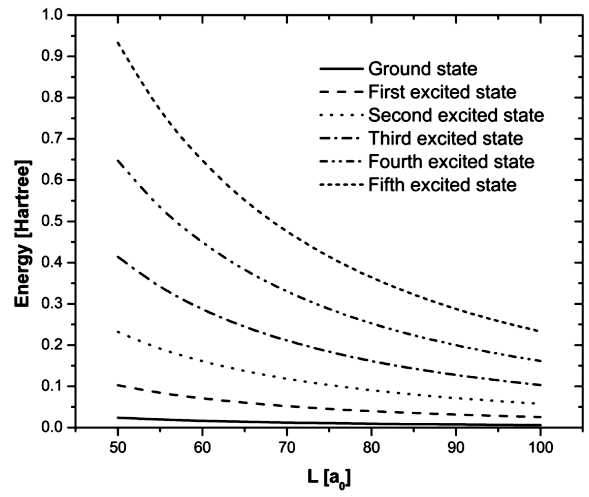Figure 1 Energy for an electron with position-dependent effective mass inside a semiconductor with L width, considering α = 0 and β = -1 in the von Roos operator, Eqs. (13,14)

The energy values obtained when solving the Schrödinger equation can be larger or smaller compared to those that would have been obtained considering an average effective mass for the electron; the energy, thereby, can be underestimated or overestimated 38,39. To verify this result, the average mass for each value of L width was estimated using Eq. (4), finding, for any value of the width, an average effective mass of 0.07633 and with this mass the energies corresponding to the states and values of L cited in Table I. Results are shown in Table II and Fig. 2.

Table II Energy for an electron with an average effective mass of 0.07633 inside a semiconductor with L width, considering α = 0 and β = -1 in the von Roos operator, Eqs. (13,14)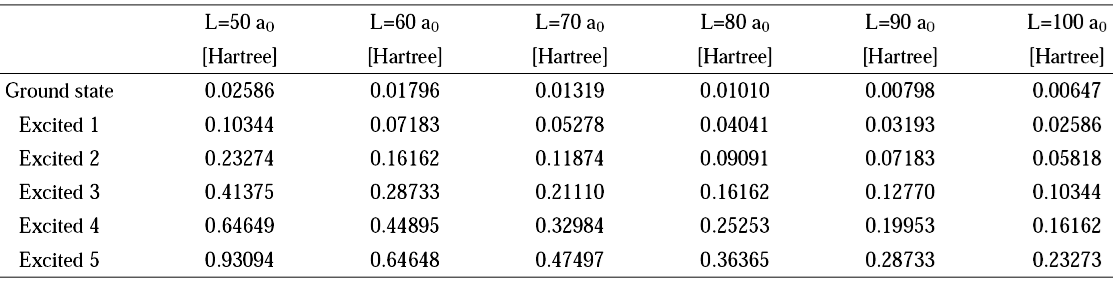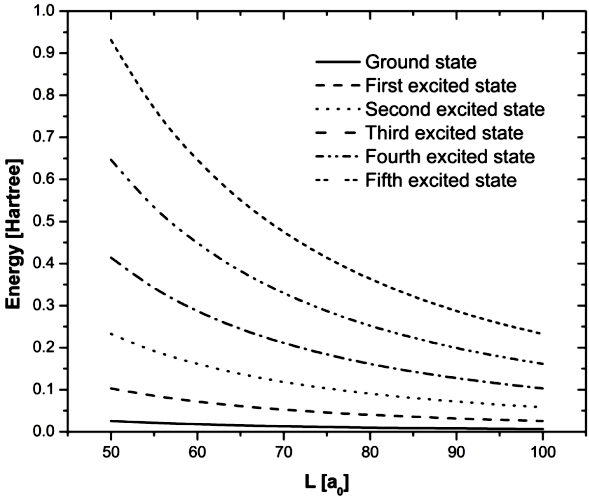Figure 2 Energy for an electron with an average effective mass of 0.07633 inside a semiconductor with L width, considering α = 0 and β = -1 in the von Roos operator, Eqs. (13,14)

In Table III a comparison between ground state energy, in atomic units, for values of L between 100 and 1000 a0, considering α = 0 and β = -1 in the von Roos operator and an effective mass of 0.07633 is shown. It can be observed that for this interval, the energies obtained considering an average value for the mass are higher to those obtained when a position-dependent mass is used. Figure 3 shows how as L increases, the difference between the energies decreases.

Table III Comparison of the ground state energy for an electron considering a position-dependent mass and an average effective mass of 0.07633.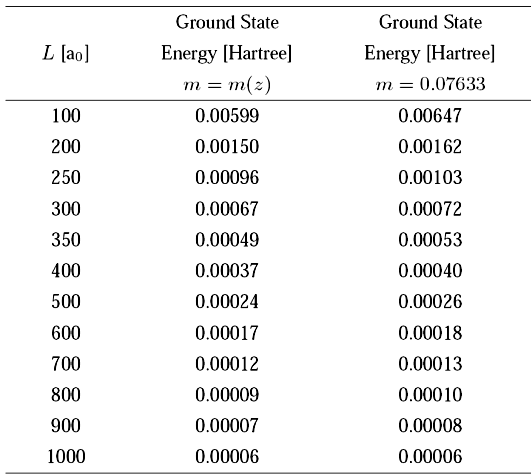Figure 3 Ground state energy for an electron considering a position-dependent mass and an average effective mass of 0.07633.

In another case we considered α = 1 to obtain from the constraint Eq. (14) the value β = -3, and according to these values, the Schrödinger Eq. (13) can be written as

-12(2L)1/2(m(z)1m3(z)m(z))j=1Najsin(jπzL)+(2L)1/2V(z)j=1Najsin(jπzL)=E(2L)1/2j=1Najsin(jπzL). (17)

As we did in the previous case, using the linear combination of the wave function for an electron in an infinite rectangular well (9, 10), the element Hi,j of the Hamiltonian matrix can be written as

Hi,j=12(2L)0L1m3(z){[m(z)]2sin(iπzL)sin(jπzL)+m[m(z)](jπL)sin(iπzL)cos(jπzL)×m[m(z)](iπL)cos(iπzL)sin(jπzL)+m2(z)(ijπ2L2)cos(iπzL)cos(jπzL)}dz+(2L)0Lsin(iπzL)V(z)sin(jπzL)dz (18)

The solution of the Schrödinger equation for this case provided us with the energies shown in Table IV and Fig. 4.

Table IV Energy for an electron with position-dependent effective mass inside a semiconductor with L width, considering α = 1 and β = -3 in the von Roos operator, Eqs. (13,14)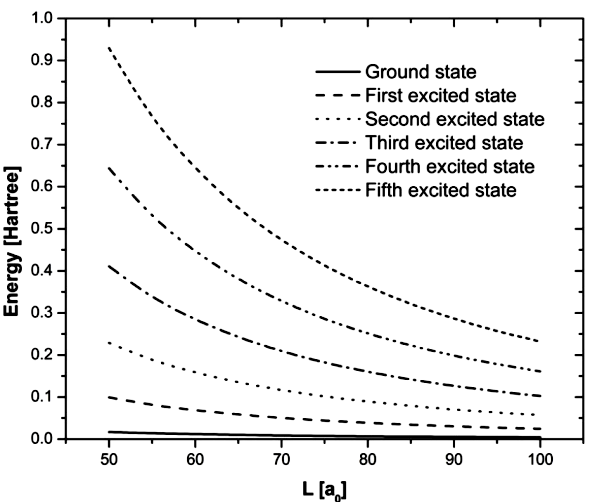Figure 4 Energy for an electron with position-dependent effective mass inside a semiconductor with L width, considering α = 1 and β = -3 in the von Roos operator, Eqs. (13,14)

Another case in which we estimated the energies was where α = 2 and β = -5, thus, the Schrödinger equation for the electron with position-dependent mass, Eq. (13), can be written as

12(2L)1/2(m2(z)1m5(z)m2(z))j=1Najsin(jπzL)+(2L)1/2V(z)j=1Najsin(jπzL)=E(2L)1/2j=1Najsin(jπzL) (19)

With the linear combination of wave functions (9,10), the element Hi,j of the Hamiltonian matrix can be written as

Hi,j=12(2L)0L1m5(z){[m2(z)]2sin(iπzL)sin(jπzL)+m2[m2(z)](jπL)sin(iπzL)cos(jπzL)+m2[m2(z)](iπL)cos(iπzL)sin(jπzL)+m4(z)(ijπ2L2)cos(iπzL)cos(jπzL)}dz+(2L)0Lsin(iπzL)V(z)sin(jπzL)dz. (20)

The solution of the Schrödinger equation for this case provided us with the energies shown in Table V and in Fig. 5.

Table V Energy for an electron with position-dependent effective mass inside a semiconductor with L width, considering α = 2 and β = -5 in the von Roos operator, Eqs. (13,14)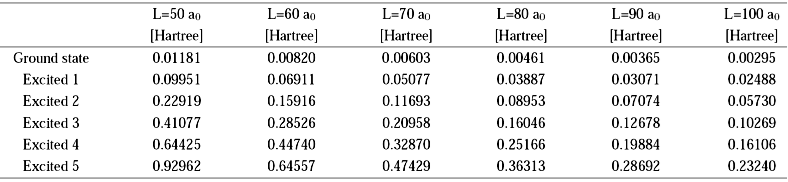Figure 5 Energy for an electron with position-dependent effective mass inside a semiconductor with L width, considering α = 2 and β = -5 in the von Roos operator, Eqs. (13,14)

Analyzing the energy from ground state and excited state for different values of the parameters α and β, it can be seen that for a specific value for those parameters the energy has a minimum value for any value of L. Table VI shows the ground state energies and the first three excited states, respectively, for different values of the parameters and L = 100.00 a0. Figure 6 shows how the energy for an α value varies.

Table VI Energy for an electron on the ground state, first excited state, second excited state and third excited state, considering L = 100:00 a0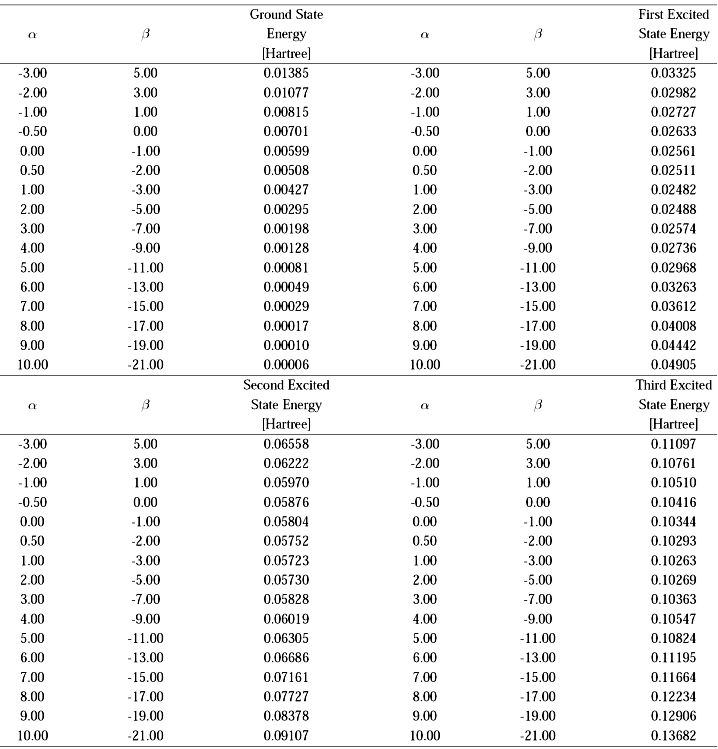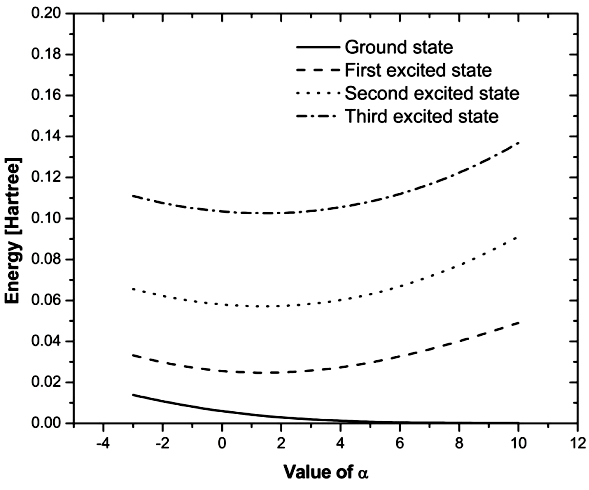Figure 6 Energy for an electron on the ground state, first excited state, second excited state and third excited state, considering L = 100:00 a0

Figure 6 reveals how for the ground state, an α value for which the energy has a minimum value is not identified, (see Table VI). On the other hand, in the case of excited states it can be observed that the energy has a minimum value for specific values of α and β parameters, thus, for the first excited state the energy will have a minimum value of 0.02474 Hartree for α = 1.43098 and β = -3.86196, while the energy of the second excited state has a minimum value of 0.05715 Hartree for α = 1.42054 and β = -3.84108, and the third excited state has a minimum value of 0.10255 Hartree for α = 1.43190 and β = -3.86380. Also considering a value of L = 500.00 a0, energy calculations were made as a function of α and β, finding a similar behavior, so that for the first excited state a minimum energy of 0.00099 Hartree for α=1.43098 and β = -3.86196 was found; for the second excited state a minimum energy of 0.00229 Hartree is found, when α = 1.42054 and β = -3.84108; and for the third excited state a minimum energy equal to 0.00410 Hartree is found for α = 1.43190 and β = -3.86380. Figure 6 also reveals how, for a given value of size L of the AlxGa1-xAs structure, the electron energy depends on the value of the α and β, parameters in the von Roos operator; this behavior is known in the literature 40,41,42 as an ordering ambiguity. In this regard, S.H. Mazharimousavi 40 notes that the general consensus is that there is not a specific value for α and β,, and he proposes an operator for the kinetic energy where there is a superposition of the α, β, and γ parameters that appear in the von Roos operator. Moreover, V.C. Ruby et al41 note that the consideration of different values for α, β, and γ causes that from the von Roos kinetic energy operator rises some terms that lead to an effective potential. However, it is possible to find some values for a best fit with results obtained in the laboratory 42.

5. Conclusions

According to consulted references, the concept of position-dependent mass is studied in various fields of physics, but it seems its use is more extensive in semiconductor theory due to what is known as effective mass. Working with a position-dependent mass in the Schrödinger equation, a special form for the operator of kinetic energy in the Hamiltonian must be used. This form can be deduced from the operator proposed by O. von Roos which is characterized by three parameters: α, β, γ, which can have integer or real values, subject to a constraint rule.

The existence of a position-dependent mass must be linked to a potential. In our study we have worked with the von Roos operator based on the study of an electron with position-dependent effective mass inside a semiconductor. Considering that the semiconductor has been deposited with a variable concentration on a substrate, a functional relationship between the effective mass of the electron and its potential energy with the concentration is established. The functional relationship defines the geometry under which the semiconductor was deposited, and on that basis, the analytical solution of the Schrödinger equation may prove complicated.

Considering that gallium-aluminum arsenide, AlxGa1-xAs was deposited, the Schrödinger equation was numerically solved in order to obtain the energies of an electron within an structure of size L, the solution was found from a linear combination of wave function of a particle inside a infinite potential well. Calculating the average effective mass and estimating the energies of the same states has allowed for a comparison of both results. The energies obtained with an average mass can be higher or lower than those obtained for a position-dependent mass, although this was not seen in our calculations, as was seen in the study of confinement of an electron in a semiconductor disk 43.

Our calculations showed that for a given value of L, the energy of the excited states has a minimum value for a determined value of the parameters in the von Roos operator. This was observed for two different values of L. Thus, given that there is no convergence in their values, there exists an ambiguity, so, generating several terms when the operator is applied on the state function, terms emerge which are being added to the potential energy leading to an effective potential. On the basis of the foregoing, the existence of a diversity of dynamic systems can be considered.

References

1. S. Adachi, J. Appl. Phys. 58 (1985) R1. [ Links ]

2. B.S. Ma et al., Appl. Phys. Lett. 91 (2007) 051102. [ Links ]

3. Z. Pan et al., Appl. Phys. Lett. 77 (2000) 1280. [ Links ]

4. X. Mei et al., Appl. Phys. Lett. 82 (2003) 967. [ Links ]

5. H. Han, D.V. Forbes and J.J. Coleman, IEEE J. Quantum Electron. 31 (1995) 1994. [ Links ]

6. C. Bin, G. Kang-Xian, W. Rui-Zhen, Z. Yun-Bao and L. Bin, Chin. J. Phys. 47 (2009) 326. [ Links ]

7. J.S. Rojas-Ramírez et al., J. of Appl. Phys. 104 (2008) 124304. [ Links ]

8. N. Aquino, G. Campoy and H. Yee-Madeira, Chem. Phys. Lett. 296 (1998) 111. [ Links ]

9. F. Arias de Saavedra, J. Boronat, A. Polls, A. Fabrocini, Phys. Rev. B 50 (1994) 4248. [ Links ]

10. S. Choi, K.M. Galdamez and B. Sundaram, Phys. Lett. A 374 (2010) 3280. [ Links ]

11. K.B. Wolf, Geometric optics on phase space, first ed. (Springer-Verlag, Berlin Heildelebrg, 2004). [ Links ]

12. D.O. Richstone and M.D. Potter, Astrophys. J. 254 (1982) 451. [ Links ]

13. W.R. Frensley, Heterostructures and Quantum Devices, VLSI Electronics: Microstructure Science. (Academic Press, San Diego, 1994). [ Links ]

14. C. Kittel, Introduction to Solid State Physics, eight ed. (John Wiley & Sons, Hoboken, 2005). [ Links ]

15. M. Molinar-Tabares and G. Campoy -Güereña, J. Comput. Theor. Nanosci. 7 (2010) 2308. [ Links ]

16. G. Ovando, J.J. Peña and J. Morales, J. of Phys.: Conference Series 490 (2014) 012201. [ Links ]

17. C. Jirauscheck, IEEE J. of Quantum Electron. 45 (2009) 1059. [ Links ]

18. L. Dekar, L. Chetouani and T.F. Hammann, J. of Math. Phys. 39 (1998) 2551. [ Links ]

19. D.A, Kulikov, ISRN Math. Phys. 2012 (2012) 461452. [ Links ]

20. R.K. Mains, I. Mehdi and G. I. Haddad, Appl. Phys. Lett. 55 (1989) 2631. [ Links ]

21. J.J. Peña , G. Ovando , J. Morales, J. García-Ravelo and C. Pacheco-García, Int. J. of Quantum Chem. 107 (2007) 3039. [ Links ]

22. B. Gönül, O. Özer, B. Gönül and F. zgün, Mod. Phys. Lett. A 17 (2002) 2453. [ Links ]

23. C. Quesne, Ann. of Phys. 321 (2006) 1221. [ Links ]

24. R. Reeder, A. Udal, E. Velmre and P. Harrison, IEEE Xplore, Conference: Baltic Electronics Conference (2006) 1. [ Links ]

25. J. Thomsen, G.T. Einevoll and P.C. Hemmer, Phys. Rev. B 39 (1989) 12783. [ Links ]

26. S. Cruz and O. Rosas-Ortiz, J. Phys. A: Math. Theor. 42 (2009) 185205. [ Links ]

27. J.J. Peña , G. Ovando , J. Morales , J. García-Ravelo andC. Pacheco-García , Int. J. of Quantum Chem. 108 (2008) 2906. [ Links ]

28. A.D. Alhaidari, Phys. Rev. A 66 (2002) 042116. [ Links ]

29. A.R. Plastino, A. Rigo, M. Casas, F. Garcias and A. Plastino, Phys. Rev. A 60 (1999) 4318. [ Links ]

30. J. Morales , G. Ovando and J. J. Peña, AIP Conf. Proc. 1323 (2010) 233. [ Links ]

31. C. Pacheco-García , J. García-Ravelo , J. Morales andJ.J. Peña , Int. J. of Quantum Chem. 110 (2010) 2880. [ Links ]

32. O. von Roos, Phys. Rev. B 27 (1983) 7547. [ Links ]

33. T. Gora and F. Williams, Phys. Rev. 177 (1969) 1179. [ Links ]

34. Q.G. Zhu and H. Kroemer, Phys. Rev. B 27 (1983) 3519. [ Links ]

35. R.A. Morrow and K.R. Brownstein, Phys. Rev. B 30 (1984) 678. [ Links ]

36. B. Bagchi, P. Gorain, C. Quesne and R. Roychoudhury, Mod. Phys. Lett. A 19 (2004) 2765. [ Links ]

37. P. Harrison , Quantum Wells, Wires and Dots, third ed. (John Wiley & Sons, Hoboken , 2010). [ Links ]

40. S.H. Mazharimousavi, arXiv:1208.4628v1 [quant-ph] 22 Aug 2012 (2012). [ Links ]

41. C.C. Ruby, V.K. Chandrasekar, M. Senthilvelan and M. Lakshmanan, J. Math. Phys. 56 (2015) 012103. [ Links ]

42. V.A. Smagley, M. Mohammad and M. Osinski, Proceedings of SPIE, 4646 (2002) 258. [ Links ]

43. M. Molinar-Tabares, C. Figueroa-Navarro, L. Castro-Arce and J. Campos-García, J. Comput. Theor. Nanosci., 12 (2015) 4767. [ Links ]

Received: January 07, 2016; Accepted: April 13, 2016This is an open-access article distributed under the terms of the Creative Commons Attribution License